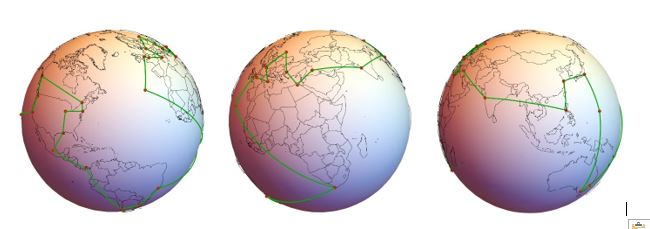Include Top

# Case Study 7 – The Travelling Salesperson Problem (TSP)Introduction: Optimizing Travel to 30 International Cities

The travelling salesperson problem (TSP) asks the following question: “Given a list of cities and the distances between each pair of cities, what is the shortest possible route that visits each city exactly once and returns to the origin city?”  The problem was first formulated in 1930 and is one of the most intensively studied problems in optimization. It is used as a benchmark for many optimization methods. The TSP has several applications such as planning, logistics, and the manufacture of microchips. Slightly modified, it appears as a sub-problem in many areas, such as DNA sequencing. In these applications, the concept city represents, for example, customers, soldering points, or DNA fragments, and the concept distance represents travelling times or cost, or a similarity measure between DNA fragments. The TSP also appears in astronomy, as astronomers observing many sources will want to minimize the time spent moving the telescope between the sources. In many applications, additional constraints such as limited resources or time windows may be imposed. (Ref.: https://en.wikipedia.org/wiki/Travelling_salesman_problem)

The books by Applegate and Cook (see Case Study 7 References) and the University of Waterloo TSP web site (http://www.math.uwaterloo.ca/tsp/index.html) are excellent resources for further study.

We will now consider a 30 City TSP problem. The data is from city distance dataset HA30 which describes the airline distances, in hundreds of miles, between 30 international cities:

https://people.sc.fsu.edu/~jburkardt/datasets/cities/cities.html.
A 30 city problem has 29!/2 = 4.42e30 possible solutions, so obviously this is not something that can be solved using the Discrete Exhaustive option! The goal is to achieve the best known solution minimum of 503 (50,300 miles), as given in Ouyang (2013):The 3D plots were created using Mathematica with code adapted from Dr. Ben Nolting: (http://datavoreconsulting.com/programming-tips/a-traveling-salesman-on-a-sphere-pitbulls-arctic-adventure/).

In DiscoverSim Version 2.1, the Genetic Algorithm has improved handling of the DSIM_IsAllDifferent function, so we will use the Genetic Algorithm to solve this TSP problem. The DSIM_IsAllDifferent function will be used in a constraint in order to ensure that the only solutions considered are unique city sequences.

Since this is a deterministic optimization problem, we technically only need a single replication, but DiscoverSim requires a minimum of 2 replications for internal consistency on stochastic problems. If the model included Input Distributions to represent uncertainty (for example if we were using travel times rather than distance), then we would typically use 1000 replications in the optimization settings.

 Summary of DiscoverSim Features Demonstrated in Case Study 7: Create Discrete Input Controls DiscoverSim Copy/Paste Cell Insert DSIM_IsAllDifferent function Create a Constraint that references the DSIM_IsAllDifferent function Run Optimization with Genetic Algorithm

# Web Demos

Our CTO and Co-Founder, John Noguera, regularly hosts free Web Demos featuring SigmaXL and DiscoverSim